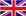English
Blog

### Transformer internal structure transformation ratio conversion method

August 12 , 2021

The current transformer is an instrument and equipment that converts a large current on the primary side into a small current on the secondary side for accurate measurement based on the basic principle of the magnetic effect of the current. It is mainly composed of a closed iron core and windings.

Transformer

The number of turns of the primary winding coil is very small, which is stringed in the route of the amount of current that must be accurately measured; the number of turns of the secondary winding coil is relatively large, and it is connected in series in the detection instrument and maintenance control loop. The secondary side of the current transformer cannot be open.

The correct method of winding and the calculation of the number of turns of the coil

You should first clarify the transformer magnification based on the size of the load, and then thread the primary wire from the transformer management center as required. Note that you cannot use the coil wound on the outer ring as the number of turns. You should use the current transformer to pass through. The number of turns in the coil is standard.

For example, a current transformer with a maximum converter ratio of 150/5 has a maximum primary rated current of 150A. If it needs to be used as a 50/5 transformer, the transmission line should be wound with 150/50=3 turns, that is, inside The ring is wound around 3 turns, and the outer ring has only 2 turns at this time. That is, if you have seen through a few turns, you only need to count the number of clear circles.

Calculation of transformation ratio and coil turns

Some current transformers have lost their factory nameplates in the application. When the customer's load changes and needs to convert the current transformer ratio, the transformer must be checked first to clarify the maximum primary rated current of the transformer, and then it must be carried out according to Calculation of transformation ratio and number of coil turns.

For example, a current transformer with a maximum primary rated current of 150A should be used as a 50/5 transformer application. The formula is converted into:

The number of turns of the core coil at one time = the current maximum primary rated current of the current transformer / the maximum primary rated current of the transformer that needs to be converted

The number of turns of the core coil at a time=150/50=3 turns

That is, it is converted into a 50/5 current transformer, and the number of turns of the core coil is 3 turns at a time. Similarly, if the transformation ratio of the original current transformer is 50/5 and the number of turns of the core coil is 3 turns, when it is to be used as a transformer of 75/5, we should first calculate the maximum primary rated current in the case of single-turn : The maximum primary rated current = the primary current in the original application × the number of turns of the original through-core coil = 50 × 3 = 150A.

The number of turns of the through-core coil after conversion to 75/5 is 150/75=2 turns. That is, when a 50/5 current transformer with 3 turns of the core through-core coil is converted to a 75/5 current transformer, the strain force of the through-core coil turns is 2 turns.

Another example is the original 50/5 current transformer with 4 turns of the core through the core, which needs to be changed into a 75/5 current transformer application. We first find the highest primary rated current of 50×4=200A, and the converted application The number of turns of the core-piercing coil should be 200/752.66 turns. When the core is actually threaded, the number of turns of the winding coil is only an integer, either 2 turns or 3 turns. No matter whether we wear 2 turns or 3 turns, there will be deviations in accurate measurement.

Therefore, when we don't know the maximum primary rated current of the current transformer, it is not possible to randomly change the ratio, otherwise it is very likely to cause a deviation in the measurement.

#### Tags

welcome to ZTC
Talk To ZTC Today To Discuss Your Need For Current Transformers,Get Standard Item, OEM/ODM Service Quote Are Available.